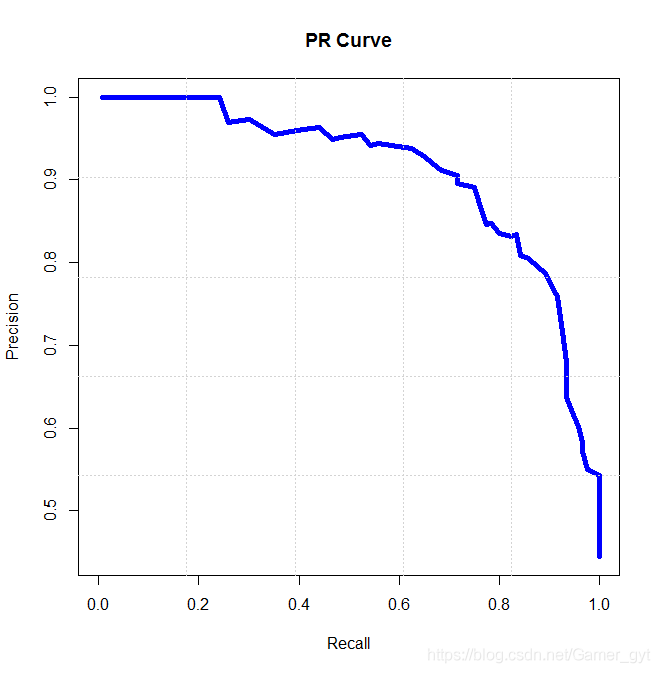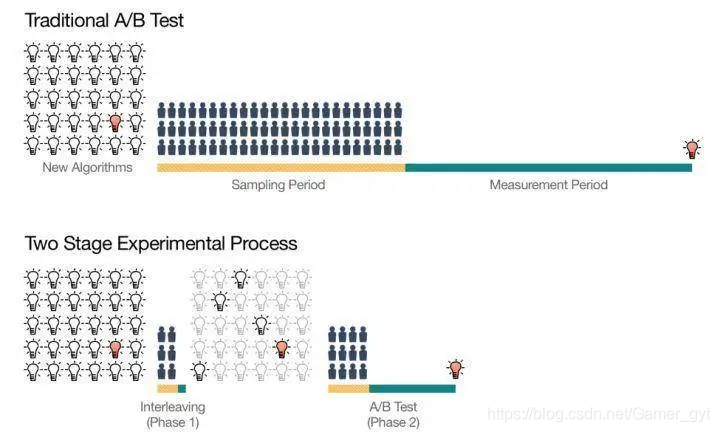# 推荐系统评估指标¶

## 场景转化指标¶

### pv点击率¶

PV(访问量)：PageView,即页面浏览量或点击量，用户每次刷新即被计算一次。

$pv点击率=\frac{pv点击}{pv}$

pv点击率是比较经典的指标，计算用户每次进入页面的情况。他能粗略衡量转化的效果，但是它缺点也比较明显：少数用户贡献大量点击会影响这个指标的作用效果。

### uv点击率¶

UV(独立访客)：Unique Visitor,访问您网站的一台电脑客户端为一个访客。00:00-24:00内相同的客户端只会被计算一次。

$uv点击率=\frac{点击uv}{整个产品的uv}$

### 曝光点击率¶

$曝光点击率=\frac{点击量}{曝光次数}$

### uv转化率¶

$uv转化率=\frac{点击uv}{进入uv}$

uv转化率尤其衡量入口型产品，比如视频app的首页，如果用uv点击率去衡量就不太科学，因为一般用户在首页点击某个视频后，会进入下一层页面深度消费，很少会返回首页继续消费，这个用户已经被转化，但不再贡献点击，所以uv转化率更加合理。

### 人均点击次数¶

$人均点击次数=\frac{点击量}{点击uv}$

## 消费满意度¶

### 留存率¶

$留存率=\frac{x日后仍活跃的用户数}{x日前的用户数}$

### 播放完成率¶

$播放完成率=\frac{播放时长}{视频时长}$

## 离线评估验证方法¶

### Holdout检验¶

Holdout检验是基础的离线评估方法，它将原始的样本集合随机划分为训练集和验证集两部分，比如70%训练集，30%测试集（但现在很多机器学习框架、深度学习框架中都增加了验证集，即将整个数据集分成三份，70%训练集，10%验证集，20%测试集）。

Holdout检验的缺点也很明显，即在验证集上计算出来的评估指标与训练集和测试机的划分有直接关系，如果仅进行少量Holdout检验，则得到的结论存在很大的随机性（在划分数据集的时候尽量保证其随机性）。

## 离线评估的主要指标¶

### 准确率¶

$accuracy=\frac{n_{correct}}{n_{total}}$

$$n_{correct}$$为被正确分类的样本个数，$$n_{total}$$为总样本个数，准确率是分类任务中比较直观的评价指标，但其优缺点也明显。

• 解释性强

#### 缺点¶

• 类别分布不均匀时，占比大的类别往往成为影响准确率的主要因素（极端的情况比如正样本 1%，负样本 99%时）

### 精确率和召回率¶

• 精确率（Precision）是分类正确的正样本个数占分类器判定为正样本的样本个数的比例

• 召回率（Recall）是分类正确的正样本个数占真正正样本个数的比例

$F1=\frac{2*Precision*Recall}{Precision+Recall}$

### 均方根误差¶

Root Mean Square Error，RMSE 经常被用来衡量回归模型的好坏，使用点击率预估模型构建推荐系统时，推荐系统预测的其实是样本为正样本的概率，RMSE被定义为：

$RMSE=\sqrt{\frac{1}{n}\sum_{i=1}^n(y_{i}-y_{pred})}$

$$y_{i}$$是第i个样本的真实值，$$y_{pred}$$是第i个样本的预测值，n为样本的个数。

#### 缺点¶

$MAPE=\sum_{i=1}^n |\frac{y_{i}-y_{pred}}{y_{i}}|*\frac{100}{n}$

### 对数损失函数¶

LogLoss，在一个二分类问题中，LogLoss定义为：

$LogLoss=-\frac{1}{N}\sum_{i=1}^N(y_{i}logP_{i}+(1-y_{i})log(1-P_{i}))$

LogLoss 是逻辑回归的损失函数，大量深度学习模型的输出层是逻辑回归或softmax，因此采用LogLoss作为评估指标能够非常直观的反映模型损失函数的变化，站在模型的角度来讲，LogLoss非常适于观察模型的收敛情况。

### P-R曲线¶

P-R曲线的横轴是召回率，纵轴是精确率，对于一个排序模型来说，其P-R曲线上的一个点代表在某一阈值下，模型将大于该阈值的结果判定为正样本，将小于该阈值的结果判定为负样本时，排序结果对应的召回率和精确率P-R曲线下的面积（Area Under Curve， AUC）能够量化P-R曲线的优劣，AUC越大，排序模型的性能越好。

### ROC曲线¶

ROC曲线的横坐标时 False Posotive Rate（FPR， 假阳性率），纵坐标时True Positive Rate（TPR，真阳性率），FPR和TPR的计算方法如下：

$FPR=\frac{FP}{N}$
$TPR=\frac{TP}{P}$

ROC曲线的绘制和P-R曲线一样，通过不断移动模型正样本阈值生成的，ROC曲线下的面积就是AUC

### 平均精度均值¶

N=1

N=2

N=3

N=4

N=5

N=6

1

0

0

1

1

1

N=1

N=2

N=3

N=4

N=5

N=6

1

0

0

1

1

1

precision@N

1/1

1/2

1/3

2/4

3/5

4/6

AP的计算只取正样本处的precision进行平均，即 AP= (1/1 + 2/4 + 3/5 + 4/6) =0.6917

### NDCG¶

#### CG¶

CG，cumulative，是DCG的前身，只考虑到了相关性的关联程度，没有考虑到位置的因素，它是一个搜索结果相关性分数的综合，指定位置p上的CG为：

$CG_{p}=\sum_{i=1}^p rel_{i}$

$$rel_{i}$$代表i这个位置的相关度。

#### DCG¶

DCG， Discounted的CG，就是在每一个CG的结果上除以一个折损值，目的是为了让排名越考前的结果越能影响最后的结果，假设排序越靠后，价值越低，那么到第i个位置时，价值为$$\frac{1}{log_{2}(i+1)}$$，那么第i个结果产生的效益是$$rek_{i}*1/log_{2}(i+1)$$，所以DCG表达式为：

$DCG_{p}=\sum_{i=1}^p \frac{rel_{i}}{log_{2}(i+1)}$
$=rel_{i}+\sum_{i=2}^{n} \frac{rel_{i}}{log_{2}(i+1)}$

$DCG_{p}=\sum_{i=1}^p \frac{2^{rel_{i}}-1}{log_{2}(i+1)}$

#### NDCG¶

NDCG，归一化的DCG，由于搜索结果随着检索词的不同，返回的数量是不一样的，而DCG是一个累加的值，没法针对两个不同的检索结果进行归一化出力，这里是除以IDCG。

$NDCG_{p}=\frac{DCG}{IDCG_{p}}$

IDCG为理想情况下的最大的DCG的值，为： $$$IDCG_{p}=\sum_{i=1}^{REL} \frac{2^{rel_{i}}-1}{log_{2}(i+1)}$$$$其中$$|REL|$表示，结果按照相关性从大到小的顺序排序，取前个结果组成的集合，也就是按照最优的方式对结果进行排序。

### 覆盖率¶

$覆盖率=\frac{推荐的物品数}{总物品数}$

### 挖掘长尾的能力¶

1. 所有长尾推荐物品中每天有多少比例被分发出去了

2. 有多少比例的用户，推荐过了长尾物品

3. 长尾内容的转化情况和产生的商业价值

## Replay¶

### 动态离线评估方法¶

Replay方法不仅适用于几乎所有推荐模型的离线评估，而且是强化学习类模型唯一的离线评估方法。

Replay的实际实现中有一点需要特别注意的是：样本中不能包含任何「未来信息」，要避免数据穿越的现象发生

### Interleaving线上评估方法¶

2013年微软提出了Interleaving线上评估方法，被当作时线上A/B测试的预选阶段进行候选算法的快速筛选，从大量初始想法中筛选出少量优秀的推荐算法，再对缩小的算法集合进行传统的A/B测试，以侧拉他们对用户行为的长期影响。

#### A/B测试存在的统计学问题¶

A/B测试虽然是样本进行随机分配，但是难免会存在分布不均匀得情况，我们都知道二八原则，当对平台用户进行分流时，没有办法保证活跃用户也能被均分，因此一种可行的方法就是不对测试人群进行分组，而是让所有测试者都可以自由的选择要测试的物品，在实验结束时，统计每个人选择不同物品的比例，进行相关的数据统计（有点像做选择题哈哈），这种方案的优点在于：Interleaving 方法的优缺点

• 所需样本少

• 测试速度快

• 结果与A/B测试无明显差异

• 工程实现的框架较A/B测试复杂，实验逻辑和业务逻辑纠缠在一起，业务逻辑会被干扰

• Interleaving方法只是对“用户对算法推荐结果偏好程度”的相对测量，不能得出一个算法真实的表现，如果需要知道某个算法的具体指标提升，不适合使用Interleaving